1594598520

# A logistic regression from scratch

In my previous blog post we worked on artificial neural networks and developed a class to build networks with arbitrary numbers of layers and neurons. While the blog referenced to the previous notebook explaining the prerequisites, there was not yet the accompanying article, which is this blog post.

This post is also available as a Jupyter Notebook on my Github, so you can code along while reading.

If you are new to Python and Jupyter, here is a short explanation on how I manage my Python environment and packages.

A short overview of the topics we will be discussing:

1. Link between neural networks and logistic regression
2. One step back: linear regression
3. From linear to (binary) logistic regression
4. Round up

# Link between neural network and logistic regression

When we hear or read about deep learning we generally mean the sub-field of machine learning using artificial neural networks (ANN). These computing systems are quite successful in solving complex problems in various fields, examples are, image recognition, language modelling, and speech recognition. While the name ANN implies that they are related to the inner workings of our brain, the truth is that they mainly share some terminology. An ANN generally consists of multiple interconnected layers, which on itself are build using neurons (also called nodes). An example is shown in figure 1.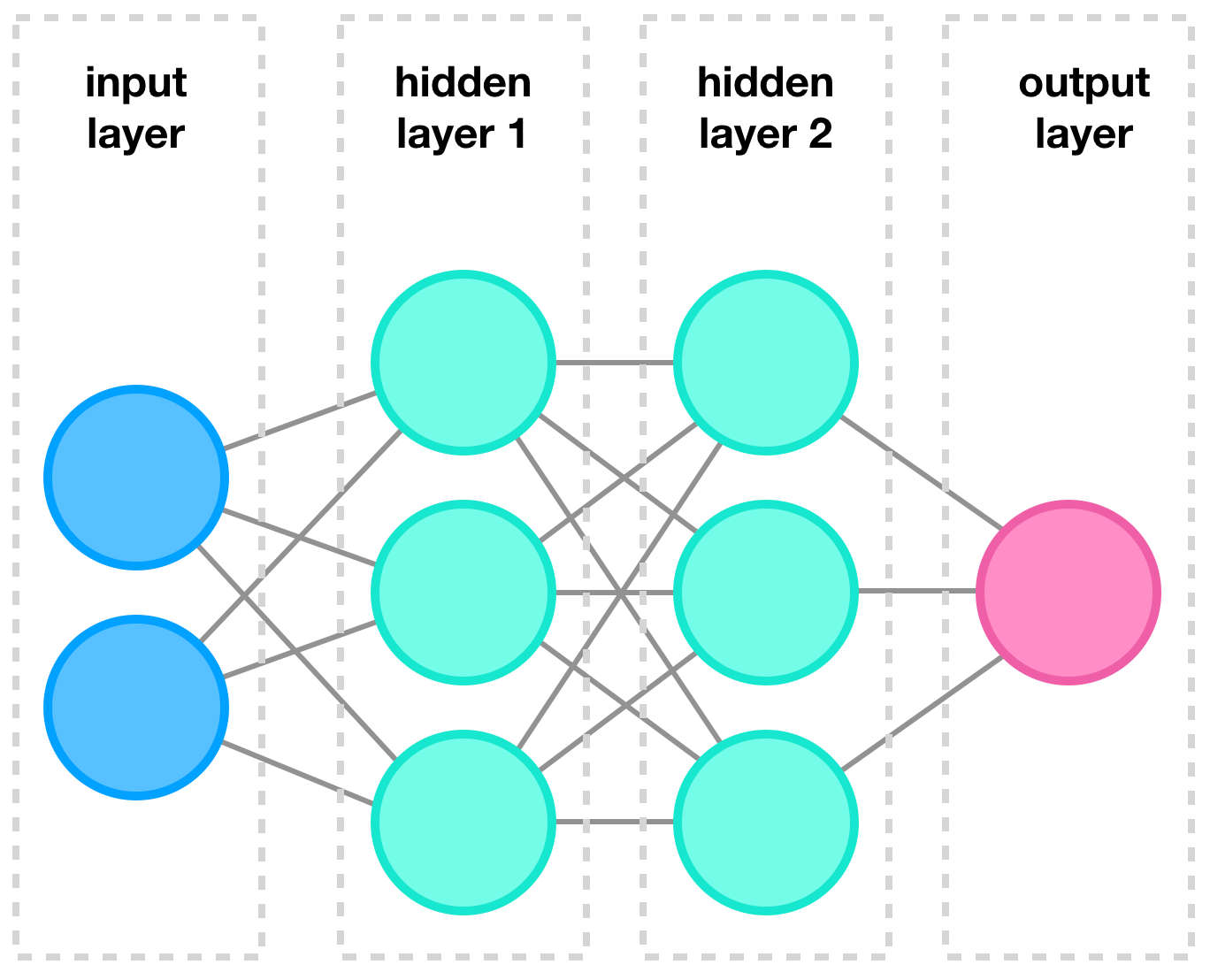Figure 1: Example of a typical neural network, borrowed from my follow up article on neural networks.

In this example, we have one input layer, consisting of four individual inputs nodes. This input layer is ‘fully connected’ to the first hidden layer, i.e. Fully connected means that each input is connected to each node. The first hidden layer is again fully connected to another ‘hidden’ layer. The term hidden indicates that we are not directly interact with these layers and these are kind of obscured to the user. The second hidden layer is on its turn fully connected two the final output layer, which consists of two nodes. So in this example we feed the model four inputs and we will receive two outputs.

Let’s now focus on a single neuron from our previous example. This neuron still is connected to all inputs, also called features. Using these features, the neuron calculates a single response (or output). A diagram of such a system is depicted in figure 2.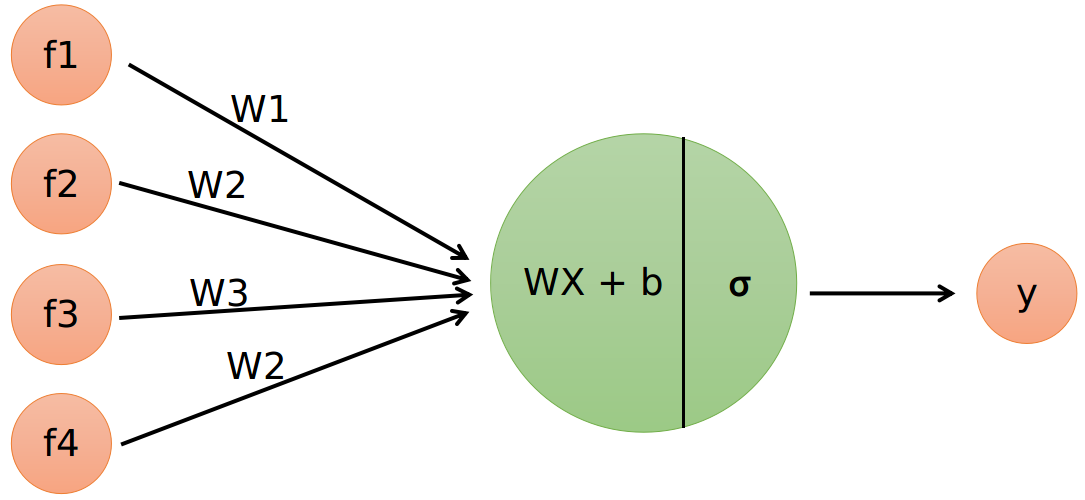Figure 2: single neuron with four input features. The neuron has two operations: a linear part and the activation function.

The input features are named 𝑓¹𝑓²𝑓³, and 𝑓⁴ and are all connected to the single neuron. This neuron executes two operations. The first operation is a multiplication of the features with a weight vector 𝑊 and adding the bias term 𝑏. The second operation is a so called activation function, indicated here by 𝜎. The output of the neuron is a probability between zero and one. The single neuron acts like a small logistic regression model and therefore, an ANN can be seen as a bunch of interconnected logistic regression models stacked together. While this idea is pretty neat, the underlying truth is a bit more subtle. There are many different architectures for ANNs and they can use various building blocks that act quite different than in this example.

The linear operation in our single neuron is nothing more than a linear regression. Therefore, to understand logistic regression, the first step is to have an idea how linear regression works. The next section will show a step by step example as a recap.

# One step back: linear regression

## What is a linear regression again?

Linear regression in its simplest form (also called simple linear regression), models a single dependent variable _𝑦 _using a single independent variable 𝑥. This may sound daunting, but was this means is that we want to solve the following equation:

``````𝑦 = a𝑥 + 𝑏
``````

In the context of machine learning𝑥 represents our input data, 𝑦 represents our output data, and by solving we mean to find the best weights (or parameters), represented by 𝑤 and 𝑏 in the linear regression equation. A computer can help us find the best values for 𝑤 and 𝑏, to have the closest match for 𝑦 using the input variable 𝑥.

For the next examples, let us define the following values for x and y:

``````𝑥 = [−2,−1,0,1,2,3,4,5]
𝑦 = [−3,−1,1,3,5,7,9,11]
``````

The values for 𝑥 and _𝑦 _have a linear relation so we can use linear regression to find (or fit) the best weights and solve the problem. Maybe, by staring long enough at these values, we can discover the relation, however it is much easier to use a computer to find the answer.

If you have stared long enough or just want to know the answer, the relation between _𝑥 _and 𝑦 is the following:

``````𝑦 = 2𝑥 + 1
``````

In the next section we will use Tensorflow to create our single neuron model and try to ‘solve’ the equation.

#machine-learning #data-science #neural-networks #data analysis

## Buddha Community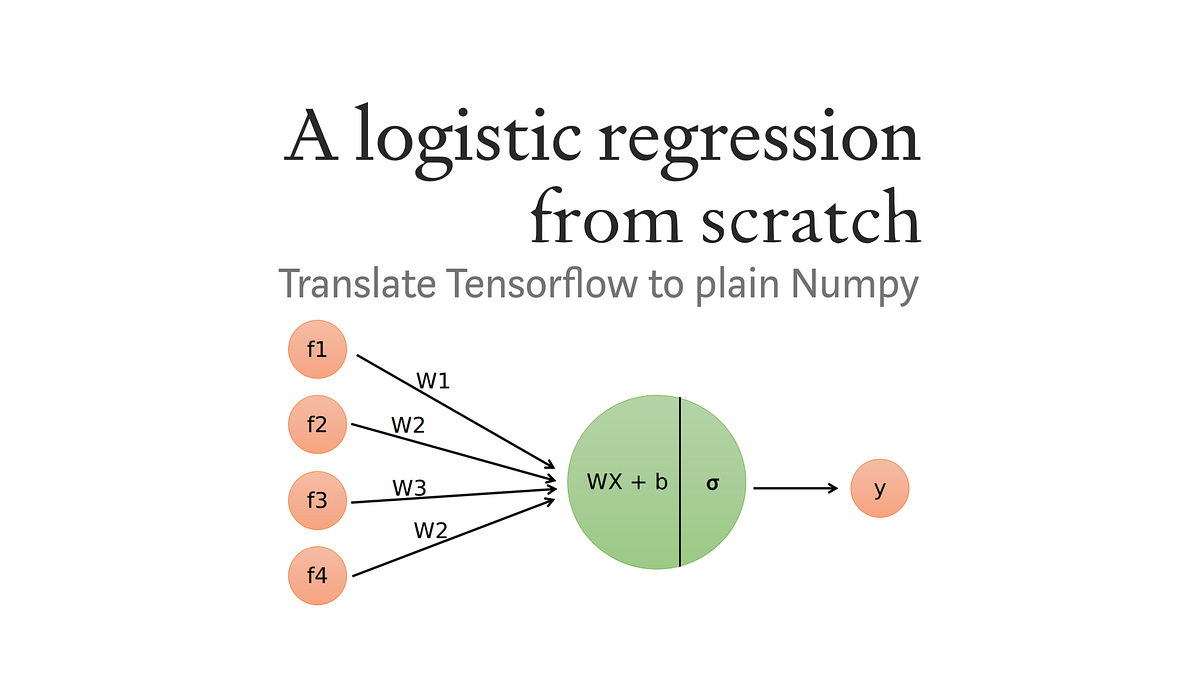1598352300

## Regression: Linear Regression

Machine learning algorithms are not your regular algorithms that we may be used to because they are often described by a combination of some complex statistics and mathematics. Since it is very important to understand the background of any algorithm you want to implement, this could pose a challenge to people with a non-mathematical background as the maths can sap your motivation by slowing you down.In this article, we would be discussing linear and logistic regression and some regression techniques assuming we all have heard or even learnt about the Linear model in Mathematics class at high school. Hopefully, at the end of the article, the concept would be clearer.

**Regression Analysis **is a statistical process for estimating the relationships between the dependent variables (say Y) and one or more independent variables or predictors (X). It explains the changes in the dependent variables with respect to changes in select predictors. Some major uses for regression analysis are in determining the strength of predictors, forecasting an effect, and trend forecasting. It finds the significant relationship between variables and the impact of predictors on dependent variables. In regression, we fit a curve/line (regression/best fit line) to the data points, such that the differences between the distances of data points from the curve/line are minimized.

#regression #machine-learning #beginner #logistic-regression #linear-regression #deep learning1600123860

## Linear Regression VS Logistic Regression (MACHINE LEARNING)

Linear Regression and Logistic Regression are** two algorithms of machine learning **and these are mostly used in the data science field.

Linear Regression:> It is one of the algorithms of machine learning which is used as a technique to solve various use cases in the data science field. It is generally used in the case of continuous output. For e.g if ‘Area’ and ‘Bhk’ of the house is given as an input and we have found the ‘Price’ of the house, so this is called a regression problem.

Mechanism:> In the diagram below X is input and Y is output value.

#machine-learning #logistic-regression #artificial-intelligence #linear-regression1592023980

## 5 Regression algorithms: Explanation & Implementation in Python

Take your current understanding and skills on machine learning algorithms to the next level with this article. What is regression analysis in simple words? How is it applied in practice for real-world problems? And what is the possible snippet of codes in Python you can use for implementation regression algorithms for various objectives? Let’s forget about boring learning stuff and talk about science and the way it works.

#linear-regression-python #linear-regression #multivariate-regression #regression #python-programming1597019820

## Introduction:

In this article, I will be explaining how to use the concept of regression, in specific logistic regression to the problems involving classification. Classification problems are everywhere around us, the classic ones would include mail classification, weather classification, etc. All these data, if needed can be used to train a Logistic regression model to predict the class of any future example.

## Context:

1. Introduction to classification problems.
2. Logistic regression and all its properties such as hypothesis, decision boundary, cost, cost function, gradient descent, and its necessary analysis.
3. Developing a logistic regression model from scratch using python, pandas, matplotlib, and seaborn and training it on the Breast cancer dataset.
4. Training an in-built Logistic regression model from sklearn using the Breast cancer dataset to verify the previous model.

## Introduction to classification problems:

Classification problems can be explained based on the Breast Cancer dataset where there are two types of tumors (Benign and Malignant). It can be represented as: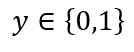where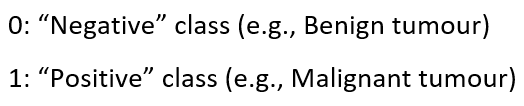This is a classification problem with 2 classes, 0 & 1. Generally, the classification problems have multiple classes say, 0,1,2 and 3.

## Dataset:

### Breast Cancer Wisconsin (Diagnostic) Data Set

Predict whether the cancer is benign or malignant

www.kaggle.com

1. Let’s import the dataset to a pandas dataframe:
``````import pandas as pd
``````

2. The following dataframe is obtained:

``````df.head()

![Image for post](https://miro.medium.com/max/1750/1*PPyiGgocvjHbgIcs9yTWTA.png)

``````

df.info()

### Data analysis:

Let us plot the mean area of the clump and its classification and see if we can find a relation between them.

``````import matplotlib.pyplot as plt
import seaborn as sns
from sklearn import preprocessing
label_encoder = preprocessing.LabelEncoder()
df.diagnosis = label_encoder.fit_transform(df.diagnosis)
sns.set(style = 'whitegrid')
sns.lmplot(x = 'area_mean', y = 'diagnosis', data = df, height = 10, aspect = 1.5, y_jitter = 0.1)
``````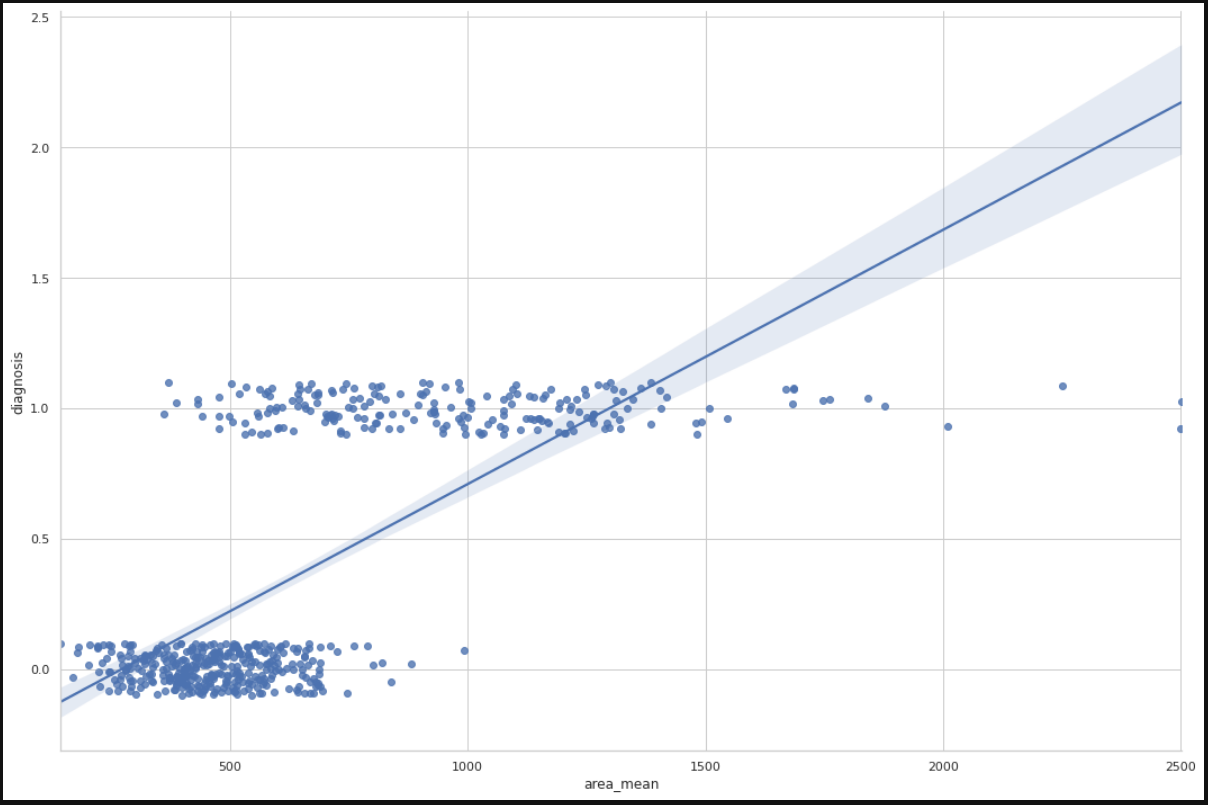We can infer from the plot that most of the tumors having an area less than 500 are benign(represented by zero) and those having area more than 1000 are malignant(represented by 1). The tumors having a mean area between 500 to 1000 are both benign and malignant, therefore show that the classification depends on more factors other than mean area. A linear regression line is also plotted for further analysis.

#machine-learning #logistic-regression #regression #data-sceince #classification #deep learning1622617140

## Logistic Regression -Beginners Guide in Python - Analytics India Magazine

Most of the supervised learning problems in machine learning are classification problems. Classification is the task of assigning a data point with a suitable class. Suppose a pet classification problem. If we input certain features, the machine learning model will tell us whether the given features belong to a cat or a dog. Cat and dog are the two classes here. One may be numerically represented by 0 and the other by 1. This is specifically called a binary classification problem. If there are more than two classes, the problem is termed a multi-class classification problem. This machine learning task comes under supervised learning because both the features and corresponding class are provided as input to the model during training. During testing or production, the model predicts the class given the features of a data point.

This article discusses Logistic Regression and the math behind it with a practical example and Python codes. Logistic regression is one of the fundamental algorithms meant for classification. Logistic regression is meant exclusively for binary classification problems. Nevertheless, multi-class classification can also be performed with this algorithm with some modifications.

#developers corner #binary classification #classification #logistic regression #logit #python #regression #scikit learn #sklearn #statsmodels #tutorial